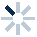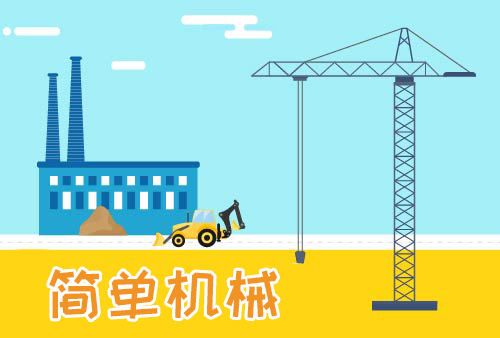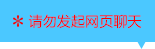| | |

||
APP端下载AndroidiPhone
|
￥40

|
13人点赞
41615人已学习
|

3天无理由退款

3天无理由退款：退款将以超级币形式退至您的超级课堂学习账户，便于您重新选购其他课程。恶意退款将被冻结账号。

• 1、容器的形状会影响液体对容器底部的压力与液体自身重力二者之间的大小关系
2、 直柱形容器中液体对容器底部的压力等于液体重力，锥形容器中液体对容器底部的压力大于液体重力，盆形容器中液体对容器底部的压力小于液体重力。所以我们可以把直柱形容器内的液体看成固体来研究
3、 求压强可以用$p=ρgh$或$p=\dfrac{F}{S}=\dfrac{G}{S}$。求压力可以用$F=G$或$F=pS$。我们要根据条件灵活选择公式，才能又快又准地求出答案
• 1、直柱形容器内的压强变化量规律是：如果用$p=ρgh$去分析，则$\Delta p=ρg\Delta h$；如果用$p=\dfrac{F}{S}=\dfrac{G}{S}$去分析，则$\Delta p=\dfrac{\Delta F}{S}=\dfrac{\Delta G}{S}=$$\dfrac{\Delta mg}{S}=\dfrac{ρ\Delta Vg}{S}$
2、 把压强的变化量和整体压强作比例，就由$\dfrac{\Delta p}{p}=\dfrac{\Delta h}{h}$。由$p=\dfrac{F}{S}$得到$\dfrac{\Delta p}{p}=\dfrac{\Delta h}{h}=\dfrac{\Delta m}{m}=\dfrac{\Delta V}{V}$，即同一个柱形容器的同种液体，压强变化量的占比，等于液体高度变化量、质量变化量、体积变化量的占比
3、 如果题目已知起始压力相同，就要先考虑压力变化量；如果题目已知起始压强相同，就要先考虑压强变化量
• 1、直柱形容器，可以直接用重力先求压力，再求压强
2、 非直柱形容器，一般要先用$p=ρgh$求压强，再用$F=pS$求压力
3、 对于由两部分直柱形容器构成的不规则形状容器，要先研究液面是否超过了下半部分，再看选用哪种方法
• 1、​感性地理解三种容器的底部受到的压力：盆形容器的侧壁会给液体斜向上的压力，所以液体对底部的压力小于液体重力；锥形容器的侧壁会给液体斜向下的压力，所以液体对底部的压力大于液体重力；直柱形容器的侧壁只给液体水平方向上的压力，所以液体对底部的压力等于液体重力
2、 无论哪种容器，它们的底部受到的压力，都等于以容器底面积为底、液体深度为高的直柱形液体的重力。这是一种重要的等效替代思想
• 1、通过两道例题，认识了两条结论：结论一：容器底的固体换成质量相同的液体后，对于盆形容器，容器底受到的压力会变小；反之，对于锥形容器，容器底受到的压力会变大
2、 结论二：质量相同的不同液体，对于同一个盆形容器，密度越大，对底部的压力和压强就越大；反之，对于同一个锥形容器，密度越大，对底部的压力和压强就越小
3、 记住这些结论，对于很多判断题和填空题，就能秒杀
• 直柱形与非直柱形容器中的液体压强综合练习

• 1Minni
• 2你好zby
• 3超级学员2350112
• 4超级学员3018273
• 5超级学员3036065
• 6超级学员3099549
• 712345
• 8超级学员3238163
• 9超级学员3419420
• 10超级学员3919003201228人在学
￥ 78 ￥ 78199795人在学
￥ 32 ￥ 32201308人在学
￥ 64 ￥ 64200614人在学
￥ 32 ￥ 32

• 0
• 点击分享有好礼
••app端下载关注微信号